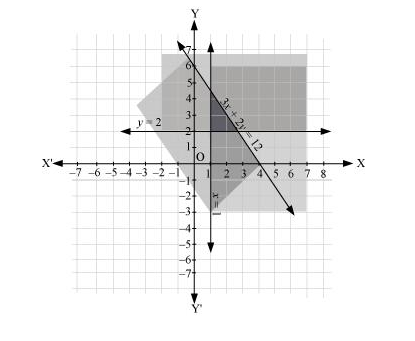# Solve the following system of inequalities graphically: 3x + 2y ≤ 12, x ≥ 1, y ≥ 2

Question:

Solve the following system of inequalities graphically: 3x + 2y  12, x  1, y  2

Solution:

3x + 2y  12 … (1)

x  1 … (2)

y  2 … (3)

The graphs of the lines, 3x + 2y = 12, x = 1, and y = 2, are drawn in the figure below.

Inequality (1) represents the region below the line, 3x + 2y = 12 (including the line 3x + 2y = 12). Inequality (2) represents the region on the right side of the line, x = 1 (including the line x = 1). Inequality (3) represents the region above the line, y = 2 (including the line y = 2).

Hence, the solution of the given system of linear inequalities is represented by the common shaded region including the points on the respective lines as follows.